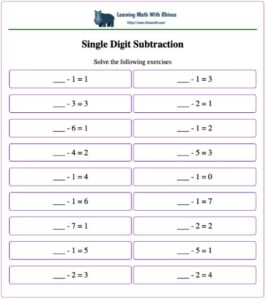## Grade 1 - Subtraction Practice

### Subtraction worksheets## Practicing Addition Equations Up To 10

• Practice Solving Subtraction Equations Up To 10
• Free to use, no registration needed
• Play Now

## Practicing Subtraction Equations Up To 20

• Practice Solving Subtraction Equations Up To 20
• Free to use, no registration needed
• Play Now

## Practicing Subtraction Equations Up To 20

• Practice Solving Subtraction Equations Up To 20
• Free to use, no registration needed
• Play Now

## Practicing Subtraction Equations Up To 50

• Practice Solving Subtraction Equations Up To 50
• Free to use, no registration needed
• Play Now

## Practicing Subtraction Equations Up To 100

• Practice Solving Subtraction Equations Up To 100
• Free to use, no registration needed
• Play Now

## Practicing Subtraction Equations Up To 100

• Practice Solving Subtraction Equations Up To 100
• Free to use, no registration needed
• Play Now

## Practicing Subtraction Equations Up To 1000

• Practice Solving Subtraction Equations Up To 1000
• Free to use, no registration needed
• Play Now